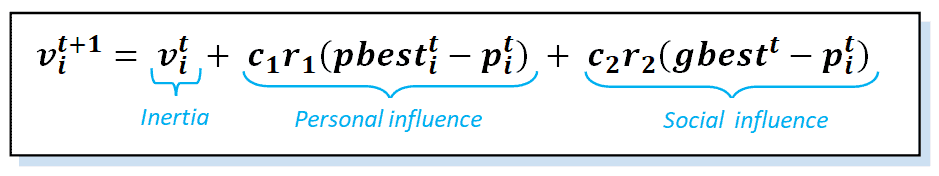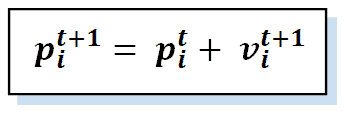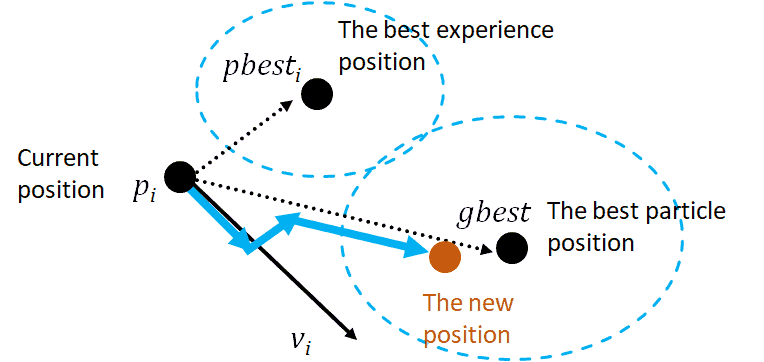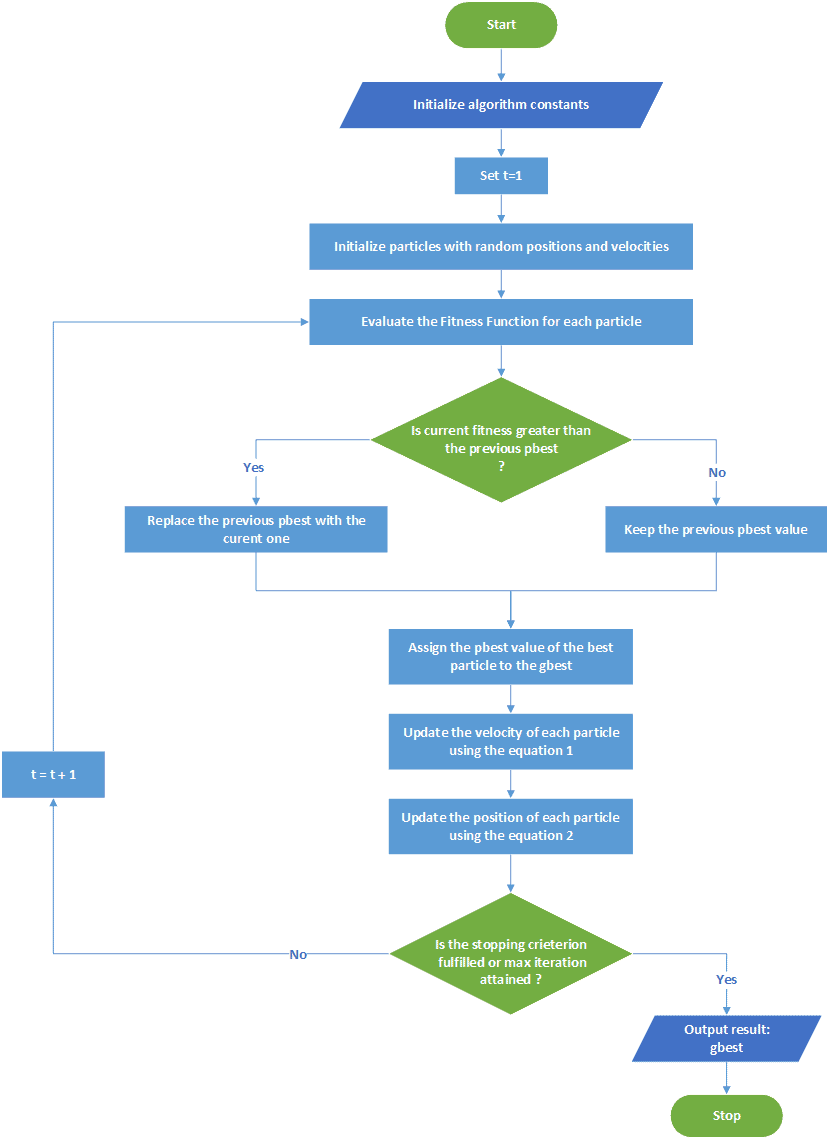## 1. Introduction

In this tutorial, we’ll understand how Particle Swarm Optimization (PSO) works. Mainly, we’ll explore the origin and the inspiration behind the idea of PSO. Then, we’ll detail the algorithm procedure. We’ll start by defining its concept and continue by mathematically modeling its parameters.

Then, we’ll continue by listing the sequence of the executing steps and representing the corresponding flowchart. Finally, we’ll present domains of applications where PSO can be used to solve real-world problems.  A finishing conclusion will summarize our tutorial.

## 2. Origin and Inspiration

The diversity in nature and its systems has driven diversity in the emerging algorithms and techniques created by imitating how nature works. We can divide them into two groups. The first one uses inputs inspired by biological entities (cells or neurons) behavior such as neural networks, genetic algorithms, and evolutionary algorithms. The second one uses inputs inspired by biological systems’ behavior, such as ants, lions, bees, etc. We call them Swarm Intelligence algorithms. In this tutorial, we’ll study the PSO algorithm and how it works.

Particle Swarm Optimization is a meta-heuristic that belongs to the category of swarm intelligence algorithms. It was first proposed by James Kennedy and Russell Eberhart in 1995 and is applied to various search and optimization problems. Let’s start by defining the three keywords in the definition. What is an optimization problem? What is a meta-heuristic? And what is swarm intelligence?

We can simply define optimization by a commonly used concept that locates the optimum solution among a set of feasible solutions. It has a fundamental role in determining some variables with certain resource limits.

Meta-heuristics are high-level procedures that aren’t dependent on the problem instance or example. We can say they are heuristics with more generalized rules than standard heuristics and can solve multiple mathematical problems.

As for swarm intelligence, we can define it as artificial intelligence that is based on the collective behavior of decentralized and self-organized systems. These systems typically comprise a population of individuals (agents) interacting locally and with their environments. The agents’ cooperation creates a collective intelligence that demonstrates great results when applied in the computer networks field. We can find many popular swarm intelligence algorithms such as ant colonies, bird flocking, fish schooling, grey wolves, ant lions, whales, etc.

## 3. Particle Swarm Optimization

Let’s look closely at the concept of work and the mathematical model of the PSO algorithm. Then, let’s show its representative flowchart.

### 3.1. Concept- How It Works

PSO is a population-based technique. It uses multiple particles that form the swarm. Each particle refers to a candidate solution. The set of candidate solutions co-exists and cooperates simultaneously. Each particle in the swarm flies in the search area, looking for the best solution to land. So, the search area is the set of possible solutions, and the group (swarm) of flying particles represents the changing solutions.

Throughout the generations (iterations), each particle keeps track of its personal best solution (optimum), as well as the best solution (optimum) in the swarm. Then, it modifies two parameters, the flying speed (velocity) and the position. Specifically, each particle dynamically adjusts its flying speed in response to its own flying experience and that of its neighbors. Similarly, it tries to change its position using the information of its current position, velocity, the distance between the current position and personal optimum, and the current position and swarm optimum.

The swarm of particles (birds) continues moving toward a promising area until getting the global optimum, which will solve the optimization problem. Following, we’ll define the mathematical models, illustrated by the used parameters and the equations, to build the PSO algorithm.

### 3.2. Parameters

The main parameters used to model the PSO are:

•: a swarm ofparticles
•: an individual in the swarm with a positionand velocity,•: the position of a particle•: the velocity of a particle•: the best solution of a particle
•: the best solution of the swarm (Global)
•: fitness function
•: acceleration constants (cognitive and social parameters)
•: random numbers between 0 and 1
•: the iteration number

### 3.3. Mathematical Models

Two main equations are involved in the PSO algorithm. The first (equation 1) is the velocity equation, where each particle in the swarm updates its velocity using the computed values of the individual and global best solutions and its current position. The coefficients ofandare acceleration factors related to the individual and social aspects.

They are known as trust parameters, withmodeling how much confidence a particle has in itself andmodeling how much confidence a particle has in its neighbors. Together with the random numbersand, they define the stochastic effect of cognitive and social behaviors:The second (equation 2) is the position equation, where each particle updates its position using the newly calculated velocity:The parameters of position and velocity are co-dependent, i.e., the velocity depends on the position and vice-versa. We can illustrate the moving particle in the following figure:### 3.4. Steps and Flowchart

After explaining the PSO principle and its mathematical model, let’s examine the PSO execution steps:

1. Initialize algorithm constants.
2. Initialize the solution from the solution space (initial values for position and velocity).
3. Evaluate the fitness of each particle.
4. Update individual and global bests (and).
5. Update the velocity and position of each particle.
6. Go to step 3 and repeat until the termination condition.

A flowchart detailing and organizing the execution steps can help us understand the PSO method:## 4. PSO Applications

PSO is known to be advantageous in many aspects. First, it is simple to implement. Second, it is derivative-free and uses very few parameters. Third, it has an efficient global search process. That is why we can say that it has been a popular technique exploited to solve several optimization problems. Let’s dig into some examples:

1. The training of neural networks which is used to identify Parkinson’s disease, extract rules from fuzzy networks, or recognize images
2. The optimization of electric power distribution networks
3. Structural optimization, where the construction industry targets the optimal shape, size, and topology during the design process
4. System identification in biomechanics

## 5. Conclusion

In this tutorial, we studied PSO, a well-known swarm intelligence method to solve optimization problems in different domains. We explained the origin and the natural inspiration of the algorithm. Then, we defined the mathematical equations and parameters adopted to model the algorithm.

After giving an illustrative flowchart of the PSO execution, we finish the tutorial by presenting some examples of the algorithm applications.